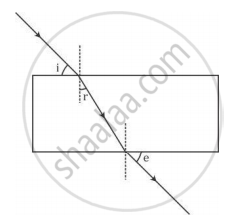# A student traces the path of a ray of light passing through a rectangular glass slab and marks the angle of incidence i, angle of refraction r and angle of emergence e, as shown. - Science

A student traces the path of a ray of light passing through a rectangular glass slab and marks the angle of incidence i, angle of refraction r and angle of emergence e, as shown.The correctly marked angle(s) is/are

(A) ∠ i only

(B) ∠ e only

(C) ∠ r only

(D) ∠ i and ∠ e

#### Solution

Angle of incidence, angle of refraction and angle of emergence, all are measured from the normal to the surface. Therefore, only angle of refraction, ∠r is marked correctly.

Hence,the correct option is C.

Concept: Refraction of Light Through a Rectangular Glass Slab
Is there an error in this question or solution?
2013-2014 (March) All India Set 3

Share